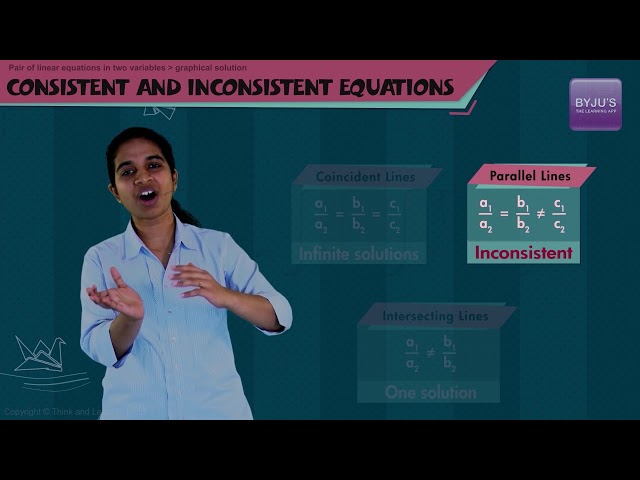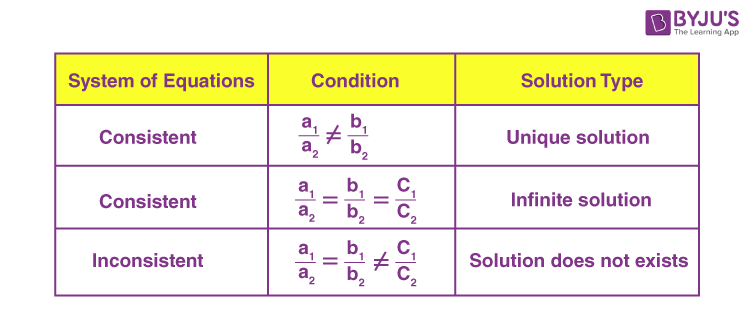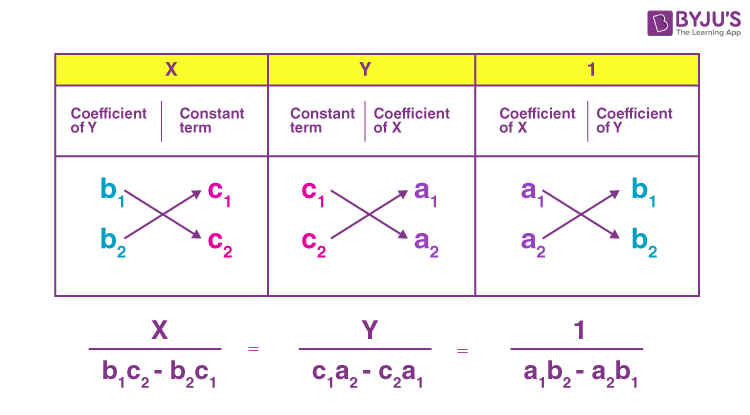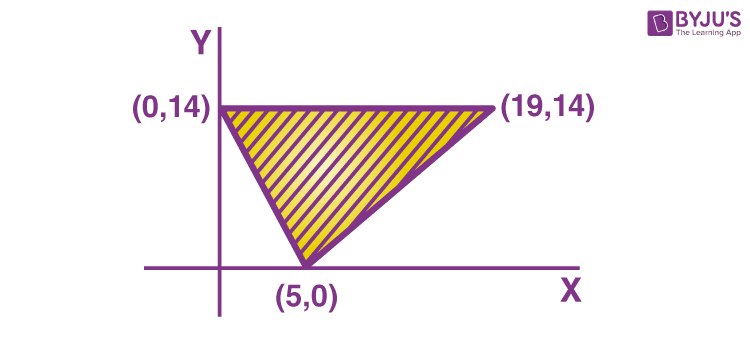Win up to 100% scholarship on Aakash BYJU'S JEE/NEET courses with ABNAT Win up to 100% scholarship on Aakash BYJU'S JEE/NEET courses with ABNAT

# Linear Equations in Algebra

Linear equations are equations having variables with power 1. ax+b = 0 is an example with one variable where x is variable, and a and b are real numbers. In this article, we will learn the definition, type of solutions, and how to solve these equations with one variable and two variables using different methods, along with examples.

Linear algebra is a subject of great breadth. Its spectrum ranges from the abstract through numerical techniques to applications. Linear algebra is used in various fields, such as fractal geometry, differential equations, difference equations, relativity, archaeology, demography and many more. In daily life, we come across various situations where we need to frame the problems into equations to solve them.

## What Is Linear Equation in Maths?

The statement of equality of two mathematical expressions involving one or more variables is known as an equation. An equation in which the highest power of the variable is always one is known as a linear equation.

Mathematically: An algebraic equation that can be written in the form ax + b = 0 or ax + by + c = 0, where a, b and c are real numbers and x and y are variables with the highest power one.

## Standard Form of a Linear Equation

Consider a, b, c, a1, a2, b1, b2 and d are real numbers, and x, y, and z are variables.

 Terms Definition Standard Form Example Linear Equations in One Variable An equation has only one variable ax + b = 0 2x – 3 = 0 Linear Equation in Two Variables An equation has only two variables ax + by + c = 0 3x + 7y + 4 = 0 Linear Equation in Three Variables An equation has three variables ax + by + cz + d = 0 x + 7y + 4z -1 = 0 Linear Equations with Fractions An equation containing fractional terms x/a = c 2x/3 = 4 Slope Intercept Form An equation in the form: y = mx + c y = mx + c, where ‘m’ = slope and ‘c’ = y-intercept y = 3x + 10 Point Slope Form Equation passing through a point (x1, y1). y − y1 = m(x − x1) where m = slope y – 3 = 10(x – 5); A line passing through point (3, 5) Pair of Linear Equations in Two Variables Set of two or more linear equations containing the same number of variables Pair of 2 equations: a1x + b1y + c1 = 0 and a2x + b2y + c2 = 0 3x + 4y = 4 and 5x + 7y = 3

In general:

 A general system of m linear equations with n unknowns: a11x1 + a12x2 + ….+ a1nxn = b1 a21x1 + a22x2 + ….+ a2nxn = b2 . . . am1x1+am2x2+….+amnxn = bm

Where x1, x2, x3,…..,xn variables and b1, b2,…., bm constant terms and a11, a12,…….,amn coefficients of any system of linear equations.

Recommended Video## Solution of Linear Equations

Linear equations can be used to solve any arithmetic equations and determine the exact value/root of the variable which satisfies the equation.

### Solving Linear Equation in One Variable

The basic principle used in solving any linear equation is that any operation performed on one side of the equation must also be performed on the other side of the equation. The following are important rules for solving linear equations:

• Subtraction rule
• Multiplication rule
• Division rule

## Solving Systems of Linear Equations

How to solve linear equations in two variables? Linear equations of two variables can be solved by different methods, as listed below. For the equations involving two variables, we need two equations having the same variables to find the roots.

Methods to solve:

1. Method of substitution
2. Cross multiplication method
3. Method of elimination
4. Matrix method
5. Determinant methods

Before you start solving linear equations having two variables, it is advised to check the type of solution, whether consistent or inconsistent. Follow the below chart to check the same.Related Articles:

## Linear Equations and Inequalities

A statement involving one or more variables having degree one is called a linear equation, whereas inequalities connect the algebraic expressions by inequality signs (greater than (>), less than (<), greater than equal to (≥), and less than equal to (⩽).

## Solved Problems on Linear Equations

Example 1: Solve 3x – 5 = 10 and check the answer.

Solution: 3x – 5 = 10

Transposing -5 to RHS,

3x = 10 + 5

3x = 15

Divide each side by 3, and we get

3x/3 = 15/3

x = 5

Checking:

Substituting x = 5 in the given equation.

3(5) – 5 = 10

15 – 5 = 10

10 = 10

Therefore, the result is true.

Example 2: Solve (3x + 4)/(x – 6) = 2/3.

Solution: (3x + 4)/(x – 6) = 2/3

Using cross-multiplication, we get

3(3x + 4) = 2(x – 6)

9x + 12 = 2x – 12

7x = -24

Example 3: Solve simultaneous linear equations by substitution method

2x + 3y = 25 and 3x + 2y =25

Solution:

2x + 3y = 25 …(i)

3x + 2y =25 ….(ii)

Isolate equation (i) for x

x = (25 – 3y)/2

Substituting the value of x in (ii)

3((25 – 3y)/2) + 2y = 25

(75 – 9y)/2 + 2y = 25

75 – 9y + 4y = 50

– 5y = -25

or y = 5

Now, find the value of x

(i)=> 2x + 3(5) = 25

x = 5

Therefore, x = 5 and y = 5 is the solution set.

## Linear Equations Word Problems

One helpful approach when solving any algebraic word problem is to translate the word problem into an equation and then solve the equation. Let us solve some of the problems to understand the concept.

Problem 1: Ten years ago, Sudheer was twelve times as old as Arshad, and ten years hence, he will be twice as old as Arshad will be. Find their present ages.

Solution:

Consider Sudheer’s present age is x years, and Arshad is y years older.

As per the given data,

 Arshad’s Age Sudheer’s Age Equation Present Age y x 10 years ago y – 10 x – 10 x – 10 = 12(y – 10) 10 years hence y + 10 x + 10 x + 10 = 2(y + 10)

We have two equations:

x – 10 = 12(y – 10) and

x + 10 = 2(y + 10)

Solving the above equations and finding the values of x and y,

x – 12y = -110 ….(i)

and

x – 2y = 10 or x = 10 + 2y …(ii)

Substituting the value of x in (i),

(10 + 2y) – 12y = -110

10 – 10y = -110

or y = 12

Now, (ii) implies

x = 10 + 2(12)

or x = 34

Therefore, Sudheer is 34 years old, and Arshad is 12 years old.

Problem 2: Raksha’s mother asked her to buy one candy and three pencils for Rs. 10. Again, she was asked to buy two candies and one pencil for Rs. 5 of the same kind. Find the cost of one candy and one pencil. [Apply cross multiplication method]

Solution:

Let x be the cost of one pencil, and y be the cost of one candy.

One candy and three pencils for Rs. 10 => 3x + y = 10

Two candies and one pencil for Rs. 5 => x + 2y = 5

Method for cross multiplication:Using the above formula, we have

a1 = 3, b1 = 1, c1 = -10

a2 = 1, b2 = 2, c2 = -5

=> x = 15/3 = 3

and y = 5/5 = 1

Therefore, the cost of one pencil is Rs. 3, and the cost of one candy is Rs. 1.

Problem 3: The shaded region shown in the figure is given by the equationsSolution:
From the figure, it is clear that there are 3 lines.
The line which passes from (0, 14) and (19, 14) is y = 14.
∴ In the shaded region 0 ≤ y ≤ 14.
The line which passes from (5, 0) and (0, 14) is 14x + 5y = 70.
∴ In the shaded region, 14x + 5y ≥ 70.
The line which passes from (5, 0) and (19, 14) is x − y − 5 = 0
∴ In the shaded region, x − y ≤ 5.
Thus, the inequations are 14x + 5y ≥ 70, x − y ≤ 5, y ≤ 14.

Problem 4: The number of real values of parameter k for which

$$\begin{array}{l}{{(lo{{g}_{16}}x)}^{2}}-{{\log }_{16}}x+{{\log }_{16}}k=0\end{array}$$
will have exactly one solution is

Solution:
The equation is

$$\begin{array}{l}{{(lo{{g}_{16}}x)}^{2}}-{{\log }_{16}}x+{{\log }_{16}}k=0\end{array}$$
.

Put y = log16 x

Then, y2 – y + log16 k = 0

For exactly one solution, b2-4ac = 0

1 – 4 log16 k = 0

log16 k = 1/4

k = 161/4

= 2

Problem 5: The solution set of the inequality 37 − (3x + 5) ≥ 9x − 8 (x − 3) is

Solution:
We have, 37 − (3x + 5) ≥ 9x − 8 (x − 3)

(37 − 3x − 5) ≥ 9x − 8x + 24
⇒ 32 − 3x ≥ x + 24
Transferring the term 24 to L.H.S. and the term (−3x) to R.H.S,
32 − 24 ≥ x + 3 x ⇒ 8 ≥ 4x
⇒ 4x ≤ 8
Dividing both sides by 4.
4x / 4 ≤ 8 / 4
⇒ x ≤ 2
∴ The solution set is (−∞, 2].

Problem 6: The solution set of (x)2 + (x + 1)2 = 25, where (x) is the least integer greater than or equal to x, is
Solution:

(x)2 + (x + 1)2 = 25

x2 + x2 + 2x + 1 = 25

2x2 + 2x – 24 = 0

x2 + x – 12 = 0

x = -4 or 3

When x = -4 -5 < x ≤ -4

and x = 3 ⇒ 2 < x ≤ 3

Therefore, the solution set is (-5, -4] U (2, 3].

Q1

### What do you mean by a linear equation?

A linear equation is an algebraic equation in which each term has an exponent of 1, and when graphed, the result is always a straight line.

Q2

### Give an example of a linear equation.

4x+3y = 10 is an example of a linear equation in 2 variables. Here, x and y are the variables and 4 and 3 are the coefficient of x and y, respectively.

Q3

### Give the standard form of a linear equation in one variable.

The standard form of linear equations in one variable is given by Ax + B = 0. Here, x is the variable, and A and B are real numbers.

Q4

### How do you solve linear equations in one variable?

We can solve a linear equation in one variable by shifting the variables to one side of the equation and the numeric part to the other side. For example, 5x + 3 = x + 11. We shift variables to one side and numbers to another side and solve,

5x-x = 11-3
4x = 8

So, x = 8/4 = 2.

Test your Knowledge on Linear equations